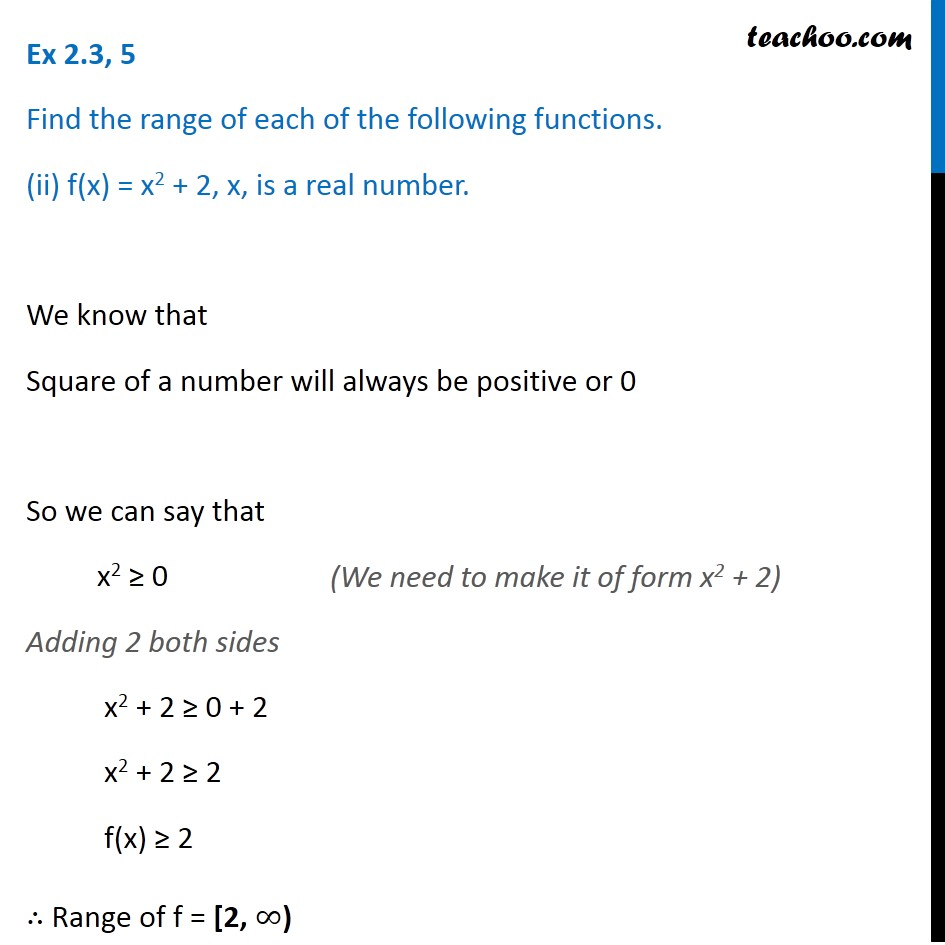1. Chapter 2 Class 11 Relations and Functions (Term 1)
2. Serial order wise
3. Ex 2.3

Transcript

Ex 2.3, 5 Find the range of each of the following functions. (ii) f(x) = x2 + 2, x, is a real number. We know that Square of a number will always be positive or 0 So we can say that x2 ≥ 0 Adding 2 both sides x2 + 2 ≥ 0 + 2 x2 + 2 ≥ 2 f(x) ≥ 2 ∴ Range of f = [2, ∞) (We need to make it of form x2 + 2)